# Algebraic Simplification of Logic Circuits

(Deutsch)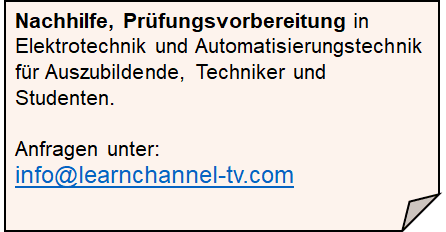Learnchannel Nachhilfe

## Simplifying logical circuits

Do you take many circuits too complex? If do so, then you can simplify them.

### Simplifying by using Boolean algebra

The following rules apply for variables which are used in logical functions. The analogy to the electrical circuit should help you to work out these rules for yourself:

#### First part: Signal a combined with a constant: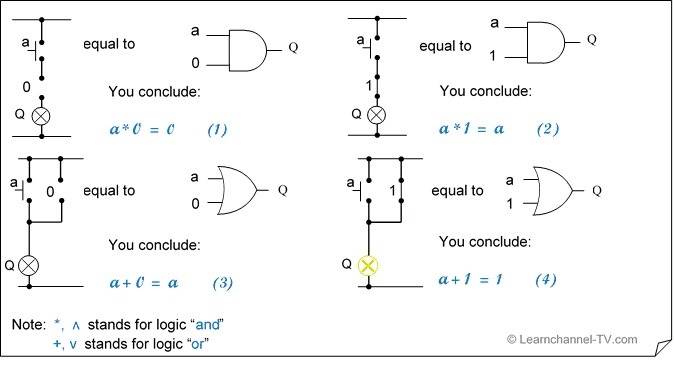Simplifying by using Boolean algebra 1

#### Second part:  Variable combined with itsself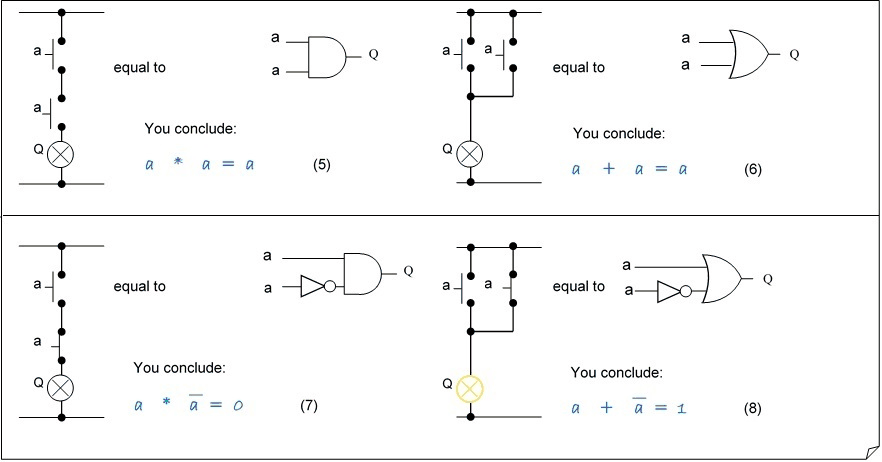Simplifying by using Boolean algebra - Variable combined with itself

#### Third part: Commutative law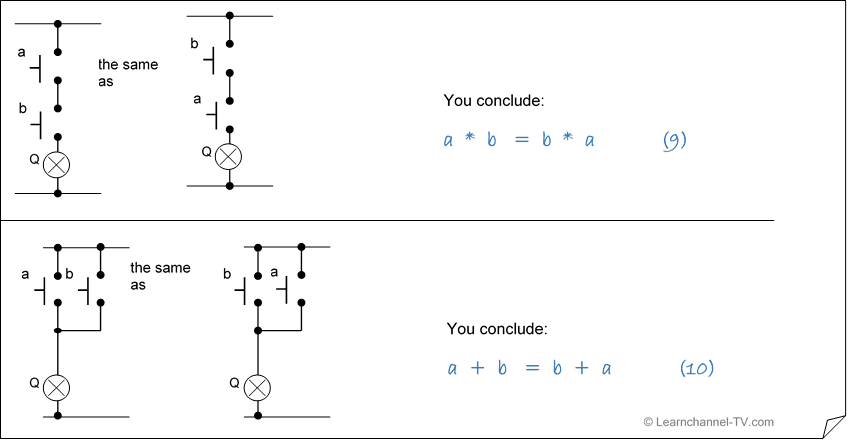Simplifying by using Boolean algebra - the Commutative law

#### Fourth part:     Associative rule

The associative rule tells you how you can summarize single variables, which are linked to each other by, AND 'or' OR '. Summarized variables are indicated by parentheses.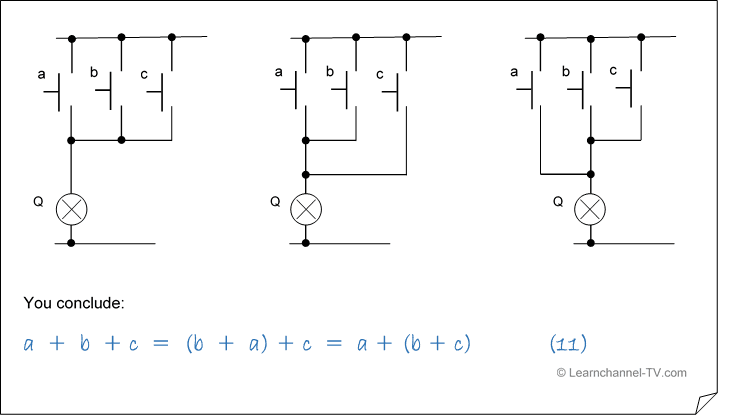Simplifying by using Boolean algebra - Associative rule

#### Fifth part:     Distributive laws

The Distributive laws specify how links relate to each other when parentheses are resolved (Law of distribution for calculating with parentheses).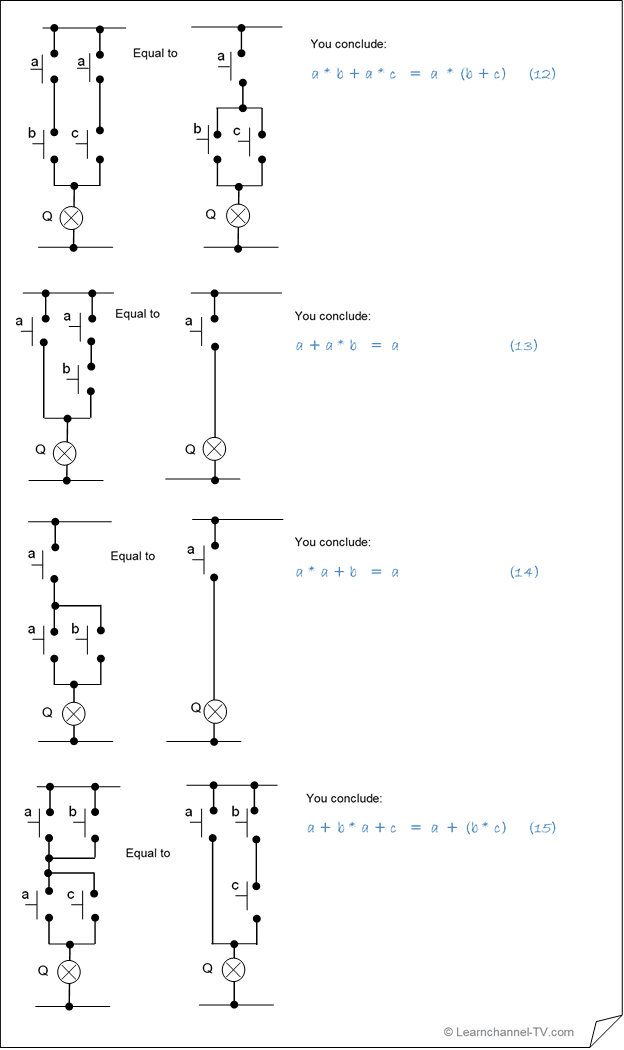Simplifying by Boolean algebra - the Distributive laws

#### Sixth part:   The rule of “de Morgan”

The De Morgan's rules are used to transform logic circuits.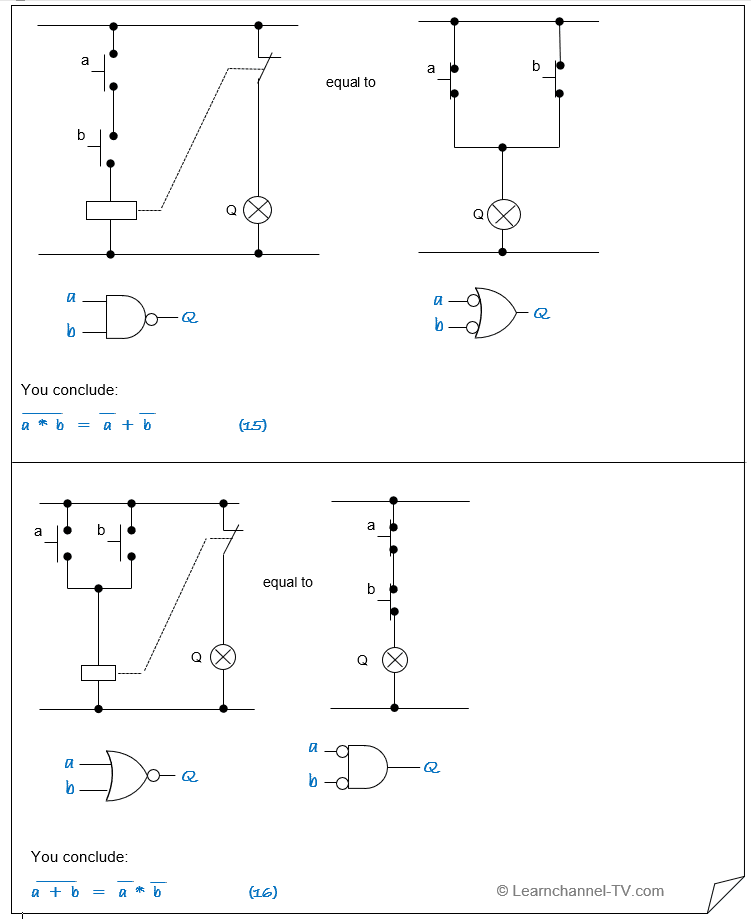Simplifying by using Boolean algebra - De Morgan's law